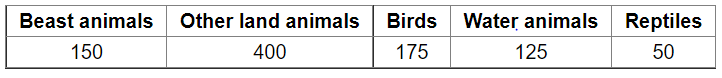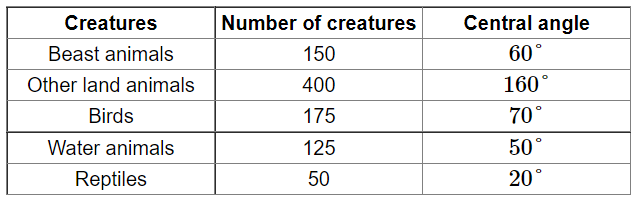# There are 900 creatures in a zoo as per list given below:

Question:

There are 900 creatures in a zoo as per list given below:Represent the above data by a pie chart.

Solution:

Total number of creatures $=900$

Central angle of each component $=\left(\frac{\text { number of creatures in each type }}{\text { total number of creatures }} \times 360\right)^{\circ}$

Calculation of central angles